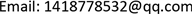Global Attractor for a Damped Wave Equation in Bounded Domain<sup> </sup>

Tingcong Zhang

School of Mathematics and Information Science, Guangzhou University, Guangzhou GuangdongReceived: Mar. 13th, 2021; accepted: Apr. 15th, 2021; published: Apr. 22nd, 2021ABSTRACT

This note considers the long-time behavior of a wave equation with damping. Based on the known results in the references, by analyzing the model, we obtain the existence of a global attractor for this kind of model.

Keywords:Bounded Domain, Damped, Wave Equation, Global Attractor1. 引言

2. 预备知识

1) K ( t ) B 是相对紧的对于所有的 t ≥ t B ；

2) s B ( t ) = sup x ∈ B ‖ S ( t ) x ‖ X < ∞ 对所有的 t ≥ t B 成立，且 s B ( t ) → 0 当 t → ∞ 。

3. 波动方程模型以及全局吸引子的构造

{ u t t + η ( − Δ ) θ u t + ( − Δ ) u = f ( u ) ,     t > 0 , x ∈ Ω , u ( x , 0 ) = u 0 ( x ) , u t ( 0 , x ) = v 0 ( x ) ,   x ∈ Ω , u ( x , t ) = 0 ,                                                               t ≥ 0 , x ∈ ∂ Ω , (3.1)

{ d d t [ u v ] + A ( θ ) [ u v ] = F ( [ u v ] ) , t > 0 , [ u ( 0 ) v ( 0 ) ] = [ u 0 v 0 ] ∈ Y (3.2)

D ( A ( θ ) ) = Y ( θ ) 1 = { [ φ ξ ] : φ ∈ X 3 2 − θ , ξ ∈ X 1 2 , A 1 − θ φ + η ξ ∈ X θ } ，对于 θ ∈ [ 0 , 1 ] ，

A ( θ ) [ φ ξ ] = [ 0 − I A η A θ ] [ φ ξ ] = [ − ξ A θ ( A 1 − θ φ + η ξ ) ] ，对 [ φ ξ ] ∈ D ( A ( θ ) ) 和 F ( [ u v ] ) = [ 0 F ( u ) ] ，

{ u t t + η A θ u t + A u = 0 , t > 0 , u ( 0 ) = u 0 , u t ( 0 ) = v 0 ,

1) 若 θ ∈ ( 0 , 1 2 ) ， − A ( θ ) 生成了一个Gevrey类 δ > 1 2 θ 的半群，因此它是可微的(参见  )，

2) 若 θ ∈ [ 1 2 , 1 ] ， − A ( θ ) 生成了一个解析半群(参见  )。在  中作者给出了 A ( θ ) 的一个分数次定义域的刻画，也就是分数阶空间 Y ( θ ) α ， α ∈ [ 1 2 , 1 ] 。

| f ( u ) − f ( u ′ ) | ≤ c | u − u ′ | ( 1 + | u | ρ − 1 + | u ′ | ρ − 1 ) (3.3)

lim sup | u | → ∞ f ( u ) u ≤ 0. (3.4)

‖ [ u ( t , u 0 , v 0 ) v ( t , u 0 , v 0 ) ] ‖ Y ≤ c + c ′ L ( [ u 0 v 0 ] ) ≤ C ( ‖ [ u 0 v 0 ] ‖ Y ) (3.5)

L ( [ w 1 w 2 ] ) = 1 2 ‖ w 2 ‖ L 2 ( Ω ) 2 + 1 2 ‖ ∇ w 1 ‖ L 2 ( Ω ) 2 − ∫ Ω ∫ 0 w 1 f ( s ) d s , w 1 , w 2 ∈ Y , (3.6)